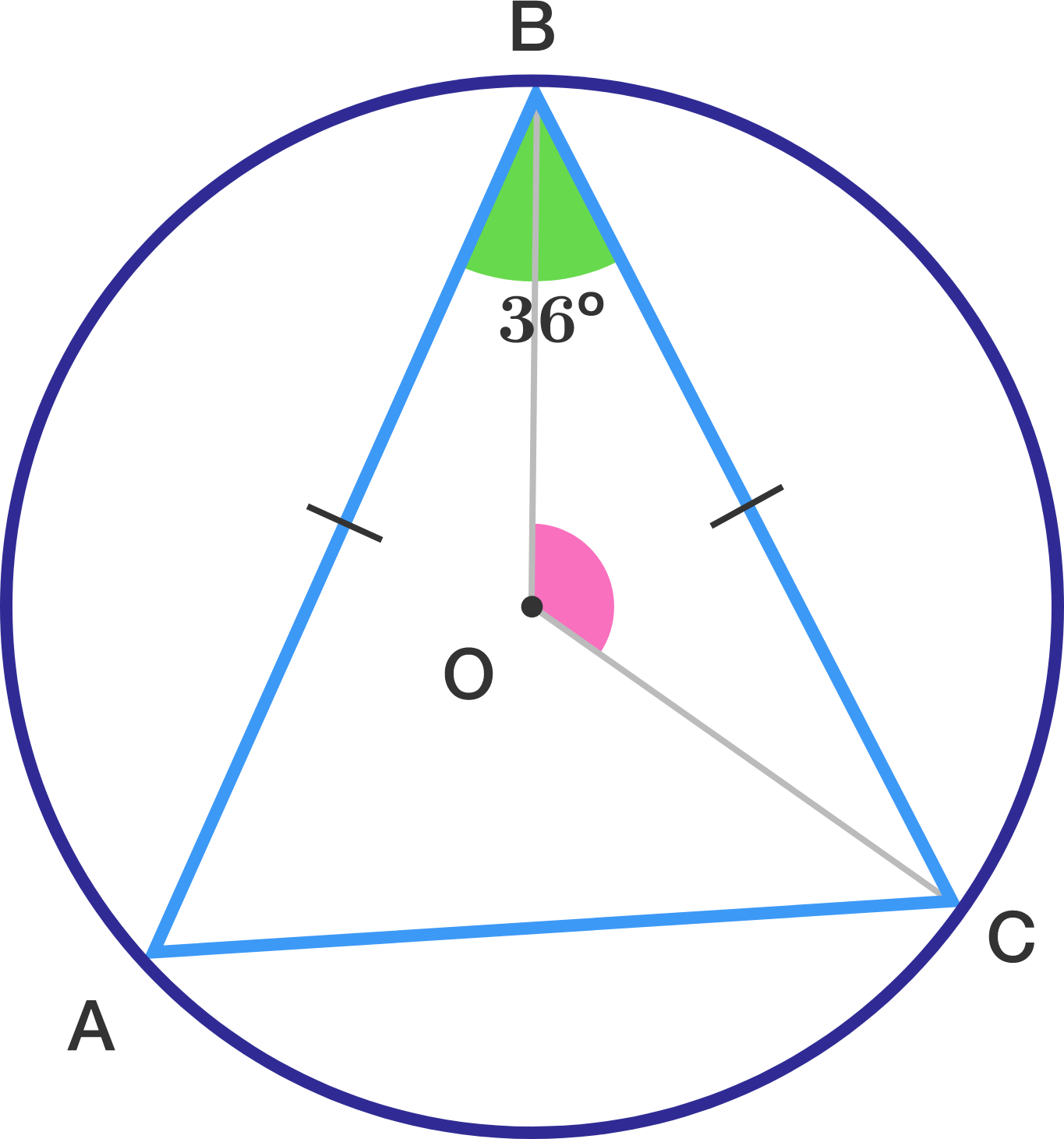Geometry Level 1In the triangle ABC sides AB and CB have equal lengths and the measure of angle ABC is equal to 36 degrees. What is the measure of angle BOC where O is the center of the circle?

Note: Figure not drawn up to scale.

×